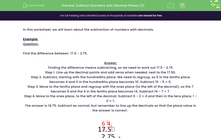Subtract Numbers with Decimal Places (2)

In this worksheet, students subtract numbers with decimal places.Key stage:  KS 2

Curriculum topic:   Number: Fractions, Decimals and Percentages

Curriculum subtopic:   Use up to Three Decimal Places

Difficulty level:Worksheet Overview

In this worksheet, we will learn about the subtraction of numbers with decimals.

Example:

Question:

Find the difference between  17.5 - 2.75.

Finding the difference means subtracting, so we need to work out 17.5 - 2.75

Step 1. Line up the decimal points and add zeros when needed, next to the 17.50.

Step 2. Subtract, starting with the hundredths place. We need to regroup, so 5 in the tenths place becomes 4 and 0 in the hundredths place becomes 10. Subtract 10 - 5 = 5.

Step 3. Move to the tenths place and regroup with the ones place (to the left of the decimal), so the 7 becomes 6 and the 4 in the tenths place becomes 14. Subtract 14 - 7 = 7.

Step 4. Move to the ones place, to the left of the decimal. Subtract 6 - 2 = 4 and then in the tens place, 1 - 0 = 1.

The answer is 14.75. Subtract as normal, but remember to line up the decimals so that the place value in the answer is correct.What is EdPlace?

We're your National Curriculum aligned online education content provider helping each child succeed in English, maths and science from year 1 to GCSE. With an EdPlace account you’ll be able to track and measure progress, helping each child achieve their best. We build confidence and attainment by personalising each child’s learning at a level that suits them.

Get started